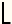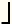# How Many Digits Does a Large Exponent Have?

## Calculating the Number of Digits in ab

 Number of Digits in ab a =    b =

For small exponential expressions such as 58, you can determine its number of digits simply by evaluating the expression and counting the digits. For instance, 58 = 390625, an integer with 6 digits.

However, it is more challenging to count the number of digits in a large exponent such as 4710000. If you try to evaluate it on a standard calculator, you will get an overflow error message since it is a number with 16721 digits.

Rather than count the number of digits in a larger exponent, it is easier to calculate their number. You can do this using logarithms and the explanation below, or the calculator at left.

### Using Log10 to Calculate the Number of Digits

The formula for the number of digits D in ab is

D =1 + Log10(ab)=1 + (b)Log10(a),

where ⌊ ⌋ is the floor function. Logarithm base-10 appears in this formula because we use a base-10 number system.

To see why the above formula works, consider the smallest 4-digit number and the smallest 5-digit number--1000 and 10000. Notice that 1000 = 103 and has 4 digits, and Log101000 = 3. Similarly, 10000 = 104 and has 5 digits, and Log1010000 = 4. The number of digits in 1000 and 10000 is one more than their logarithms.

Now consider a number between 1000 and 10000, for example, 5481. This is a number with 4 digits and Log105481 = 3.7389. The number of digits in 5481 is equal to 3.7389 rounded up to the nearest whole number.

Thus, to compute D, you take the logarithm of the number in base-10, add 1, and then round down to the nearest integer.

Example: Calculate the number of digits in 77778888.

D =1 + Log1077778888=1 + 8888Log107777= 34582.